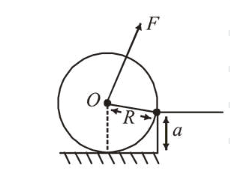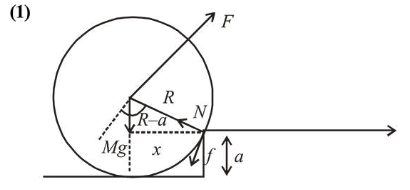# A uniform cylinder of mass M and radius R

Question:

A uniform cylinder of mass $M$ and radius $R$ is to be pulled over a step of height $a(a1.$M g \sqrt{1-\left(\frac{R-a}{R}\right)^{2}}$2.$M g \sqrt{\left(\frac{R}{R-a}\right)^{2}-1}$3.$\mathrm{Mg} \frac{a}{R}$4.$M g \sqrt{1-\frac{a^{2}}{R^{2}}}$Correct Option: 1 Solution:For step up,$F \times R \geq M g \times xx=\sqrt{R^{2}-(R-a)^{2}}$from figure$F_{\min }=\frac{M g}{R} \times \sqrt{R^{2}-(R-a)^{2}}=M g \sqrt{1-\left(\frac{R-a}{R}\right)^{2}}\$